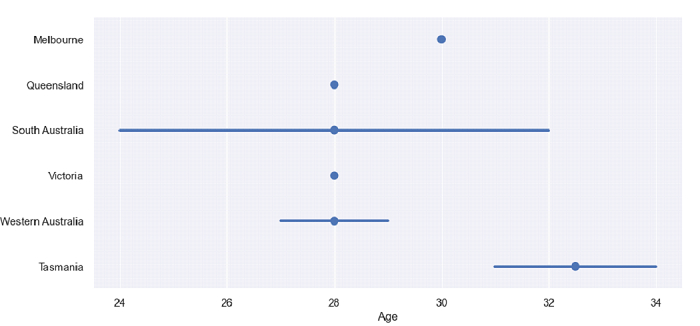# Python Pandas - Draw a set of Horizontal point plots but do not draw lines to connect points with Seaborn

Point Plot in Seaborn is used to show point estimates and confidence intervals using scatter plot glyphs. The seaborn.pointplot() is used for this. To avoid drawing lines to connect points, simply set the “join” parameter of the pointplot() method to False.

Let’s say the following is our dataset in the form of a CSV file − Cricketers.csv

At first, import the required libraries −

import seaborn as sb
import pandas as pd
import matplotlib.pyplot as plt

Load data from a CSV file into a Pandas DataFrame −

dataFrame = pd.read_csv("C:\Users\amit_\Desktop\Cricketers.csv")

Now, plot the Horizontal point plot. The “join” parameter is set as False to avoid drawing lines to connect points −

sb.pointplot(x = 'Age', y = 'Academy', data = dataFrame, join=False)

## Example

Following is the complete code −

import seaborn as sb
import pandas as pd
import matplotlib.pyplot as plt

# Load data from a CSV file into a Pandas DataFrame

sb.set_theme(style="darkgrid")

# Horizontal point plot
# join is set as false to avoid drawing lines to connect points
# join is set using the join parameter
sb.pointplot(x = 'Age', y = 'Academy', data = dataFrame, join=False)

# display
plt.show()

## Output

This will produce the following output −